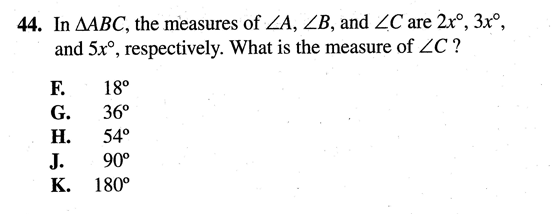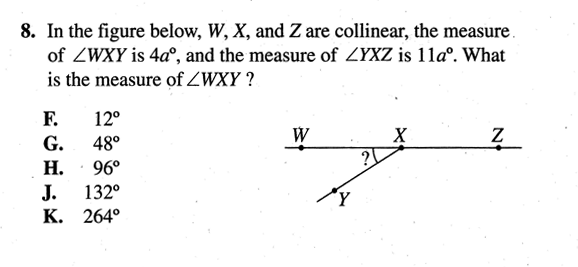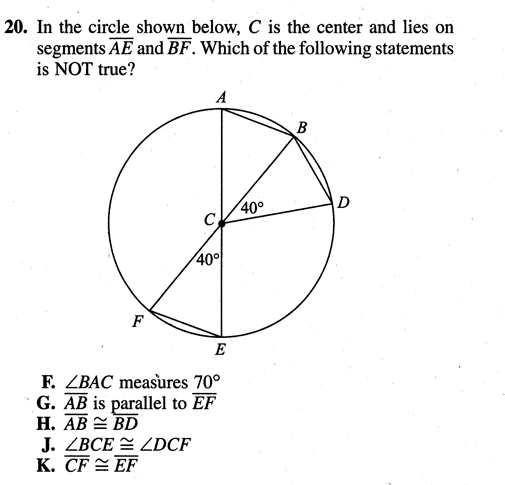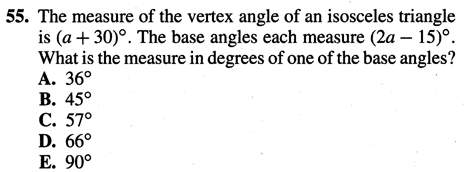Imagination is more important than knowledge... Albert Einstein

Guess is more important than calculation --- Knowhowacademy.com

Angle - Triangle, Cirlce related

For ACT
Number of Total Problems: 29.
FOR PRINT ::: (Book)

 Problem Num : 1 Type: None Section:Angle Theme:None Adjustment# : 1 Difficulty: 1Category Triangle, Cirlce related
Analysis 6a=90 therefore, 2a=30

 Problem Num : 2 Type: None Section:Angle Theme:None Adjustment# : 2 Difficulty: 1Category Triangle, Cirlce related
Analysis

 Problem Num : 3 Type: None Section:Angle Theme:None Adjustment# : 3 Difficulty: 1Category Triangle, Cirlce related
Analysis Can you guess the answer? I want you to increase the guessing power.

Solution/Answer 15a =180 what is the 4a?

 Problem Num : 4 Type: None Section:Angle Theme:None Adjustment# : 5 Difficulty: 1Category Triangle, Cirlce related
Analysis This is about isosceles triangle

 Problem Num : 5 Type: None Section:Angle Theme:None Adjustment# : 6 Difficulty: 1Category Triangle, Cirlce related
Analysis

 Problem Num : 6 Type: None Section:Angle Theme:None Adjustment# : 7 Difficulty: 1Category Triangle, Cirlce related
Analysis

 Problem Num : 7 Type: None Section:Angle Theme:None Adjustment# : 9 Difficulty: 1Category Triangle, Cirlce related
Analysis

 Problem Num : 8 Type: None Section:Angle Theme:None Adjustment# : 10 Difficulty: 1Category Triangle, Cirlce related
Analysis 5a=180 Therefore, 2a will be ...

 Problem Num : 9 Type: None Section:Angle Theme:None Adjustment# : 12 Difficulty: 1Category Triangle, Cirlce related
Analysis We need a sum to calculate the average

 Problem Num : 10 Type: None Section:Angle Theme:None Adjustment# : 12 Difficulty: 1Category Triangle, Cirlce related
Analysis